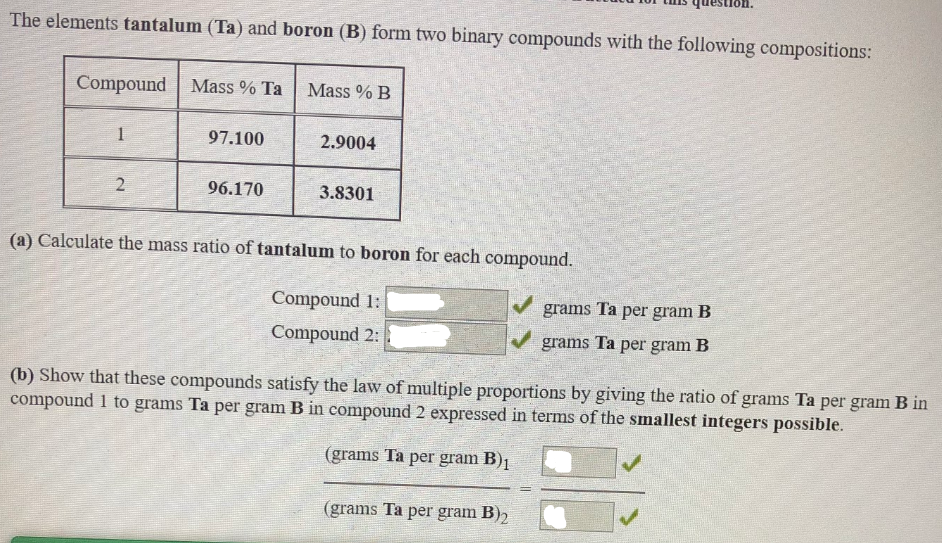# The elements tantalum(Ta) and boron (B) form two binary compounds with the following compositions: (a) Calculate the mass ratio of tantalum to boron for each compound. (b) Show that these compounds satisfy the law of multiple proportions by giving the ratio of grams Ta per gram B in compound 1 to grams Ta per gram B in compound 2 Expressed in terms of the smallest integers possible.